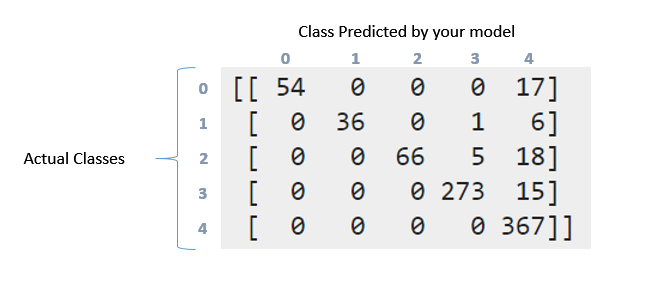2 views

I have a sentiment analysis task, for this I'm using this corpus the opinions have 5 classes (very neg,  neg, neu, pos, very pos), from 1 to 5. So I do the classification as follows:

from sklearn.feature_extraction.text import TfidfVectorizer

import numpy as np

tfidf_vect= TfidfVectorizer(use_idf=True, smooth_idf=True,

sublinear_tf=False, ngram_range=(2,2))

from sklearn.cross_validation import train_test_split, cross_val_score

import pandas as pd

X = tfidf_vect.fit_transform(df['content'].values)

y = df['label'].values

from sklearn import cross_validation

X_train, X_test, y_train, y_test = cross_validation.train_test_split(X,

y, test_size=0.33)

from sklearn.svm import SVC

svm_1 = SVC(kernel='linear')

svm_1.fit(X, y)

svm_1_prediction = svm_1.predict(X_test)

Then with the metrics, I obtained the following confusion matrix and classification report, as follows:

print '\nClasification report:\n', classification_report(y_test, svm_1_prediction)

print '\nConfussion matrix:\n',confusion_matrix(y_test, svm_1_prediction)

Then, this is the result:

Classification report:

precision-recall f1-score   support

1       1.00   0.76 0.86        71

2       1.00   0.84 0.91        43

3       1.00   0.74 0.85        89

4       0.98   0.95 0.96       288

5       0.87   1.00 0.93       367

avg / total       0.94 0.93   0.93 858

Confussion matrix:

[[ 54   0 0 0  17]

[  0 36   0 1 6]

[  0 0  66 5 18]

[  0 0   0 273 15]

[  0 0   0 0 367]]

How can I interpret the above confusion matrix and classification report. I tried reading the documentation and this question. But still can interpretate what happened here particularly with this data?. Wny this matrix is somehow "diagonal"?. By the other hand what means the recall, precision, f1score and support for this data?. What can I say about this data?. Thanks in advance guys

by (33.1k points)

You need a classification report to understand the accuracy predictions of the trained model.

Classification report must be simple, it should consist precision, recall and f1 score for each element in your test data. In Multiclass problems, you should not consider, Precision/Recall and F-Measure over the whole data, because those reports are less helpful there.

Confusion matrix, it is a detailed representation of summary of your labels. If there are 71 points in the first class (label 0), then your model was successful in predicting 54 of those correctly in label 0, but 17 were marked as label 4. There were 43 points in class 1, but 36 of them were marked correctly. Your classifier predicted 1 in class 3 and 6 in class 4.You can see the pattern this follows. Ideal classifiers with 100% accuracy would produce a pure diagonal matrix that would have all the points predicted in their correct class.

For Recall/Precision: They provide a detailed summary of the points, where your model predicted wrong.

F Measure is the harmonic mean of Precision and Recall.

Study Machine Learning Algorithms and Machine Learning Tutorials to gain more insights.